# Crate rustml [−] [src]

Library for doing machine learning with Rust.

Linear regression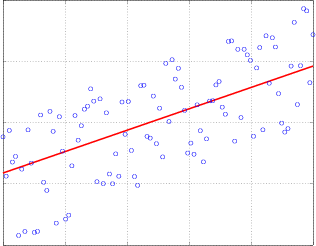MNIST database of handwritten digits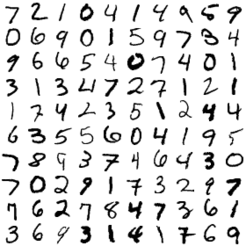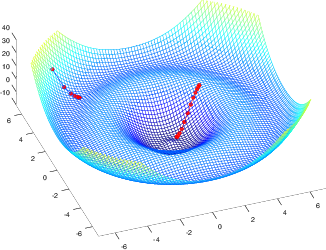Neural networks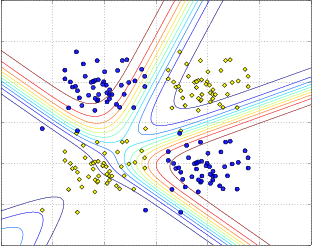Toy data: mixture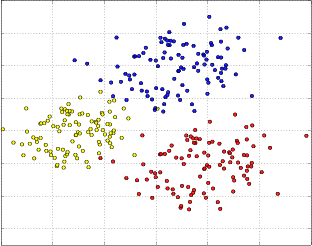Decision boundary for knn (k = 5)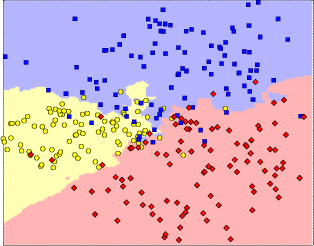# Features

(click on a link to get more details)

• highly optimized linear algebra via BLAS integration (i.e. operations on vectors and matrices)
• gradient descent with debugging capabilities (e.g. with learning curves)
• neural networks
• DBSCAN clustering algorithm
• linear regression
• optimization of linear regression with gradient descent
• classification with k-nearest neighbours
• sliding windows for arbitrary dimensions (e.g. for image processing)
• standard databases (e.g. MNIST database of handwritten digits)
• feature scaling
• video and image processing via integration of OpenCV

# Performance

When it comes to vector and matrix operations rustml makes heavy use of highly optimized numeric libraries like BLAS or ATLAS. By default CBLAS is used because it is installed on many systems by default. However, in many cases performance can be greatly improved when switching to ATLAS. For a detailed description on how to optimize the numeric computations please read the separate documentation on this topic available here.

# Machine Learning Pipelines with Rustml

The Rustml pipeline is a small and simple framework to build and configure machine learning pipelines that have been shown to be a quite powerful technique when doing machine learning. How pipelines can be created with Rustml can be seen here.

# Example how to do classifications

In the following example a simple k-nearest neighbour algorithm is used to predict the label of a vector with two features based on the examples in the matrix `m` (the training set) with their known labels stored in the vector `labels`.

```use rustml::*;

let m = mat![  // training set
1.0, 2.0;  // each row contains one example for which the label is
1.1, 2.1;  // known
2.0, 3.0;
0.9, 1.9;
2.1, 2.9
];

let labels = vec![1, 2, 2, 1, 2];

// predict the label for feature vector [1.3, 2.0]
let target =
knn::classify(
&m, &labels, &[1.3, 2.0],
3, // look at the 3 nearest neighbours to make the decision
|x, y| Euclid::compute(x, y).unwrap() // use Euclidean distance
);
assert_eq!(target, 1);```

# All examples

## Reexports

 `pub use distance::{Distance, Euclid, DistancePoint2D};` `pub use matrix::{HasNan, Similar, Matrix, IntoMatrix};` `pub use math::{Dimension, Normalization, Mean, MeanVec, Sum, Var, SumVec};` `pub use ops::{MatrixScalarOps, Ops, VectorScalarOps, VectorVectorOps, MatrixMatrixOps};` `pub use ops_inplace::{VectorVectorOpsInPlace, MatrixMatrixOpsInPlace};` `pub use gaussian::{GaussianEstimator, GaussianFunctions, Gaussian};` `pub use geometry::{Point2D};` `pub use vectors::{Linspace, VectorIO};` `pub use datasets::{mixture_builder, normal_builder};`

## Modules

 blas Bindings for BLAS/ATLAS for high performance vector and matrix operations. consts csv Functions to parse and create comma-separated values (CSV). datasets Module to easily access popular datasets often used to measure the performance of machine learning algorithms. dbscan Implementation of the DBSCAN clustering algorithm. distance Functions to compute the distance between vectors. gaussian Module to handle Gaussian distributions. geometry Collection of some common data structures. hash Hash functions. io Functions to read and write files (e.g. gzip compressed files). knn Functions to compute the k-nearest neighbours. math Module with a collection of different mathematical functions. matrix Module that contains structs and functions useful for doing matrix operations. nn Module which provides implementations of neural networks. norm Functions to compute norms of vectors. octave opencv Experimental module for image and video manipulation. ops ops_inplace Provides scalar, vector, vector-vector, vector-matrix and matrix-matrix operations. opt Module for optimization with gradient descent. regression Module for linear regression. scaling Module to scale vectors and matrices. sliding Sliding windows over strings, bytes and ranges for arbitrary dimensions. vectors Functions for vectors.

## Macros

 mat! Macro to create a matrix.